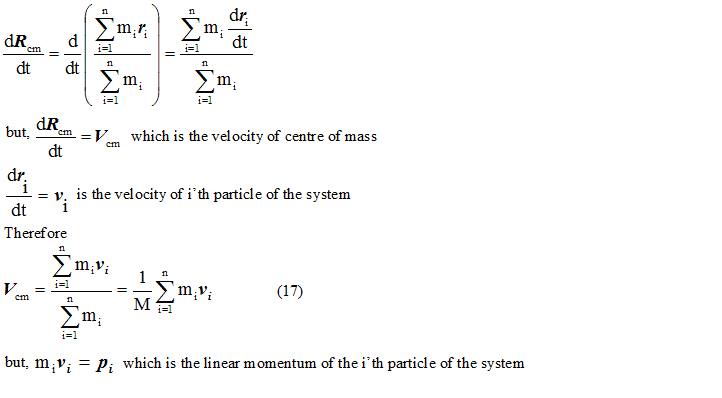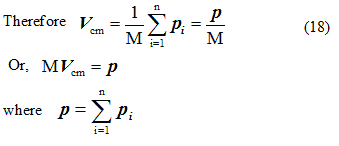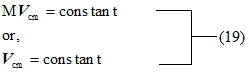# Velocity of Center Of mass

## Velocity of center of mass

• Differentiating equation $${\vec{R}_{CM}} = \frac{{\sum\limits_{i = 1}^n {{m_i}{{\vec r}_i}} }}{{\sum\limits_{i = 1}^n {{m_i}} }}$$ we getp is the vector sum of Linear Momentum of various particles of the system or it is the total linear momentum of the system.
• If no external force is acting on the system then its linear momentum remains constant. Hence in absence of external force• In the absence of external force velocity of center of mass of the system remains constant or we can say that center of mass moves with the constant velocity in absence of external force.
• Hence from equation 18 we came to know that the total linear momentum of the system is equal to the product of the total mass of the system and the velocity of the center of mass of the system which remains constant.
• Thus in the absence of the external force it is not necessary that momentum of individual particles of system like p1 , p2 , p3 . . . . . . . . . . . pn etc. remains constant but their vector sum always remains constant.

Watch this tutorial for information on How to solve Center of Mass problems